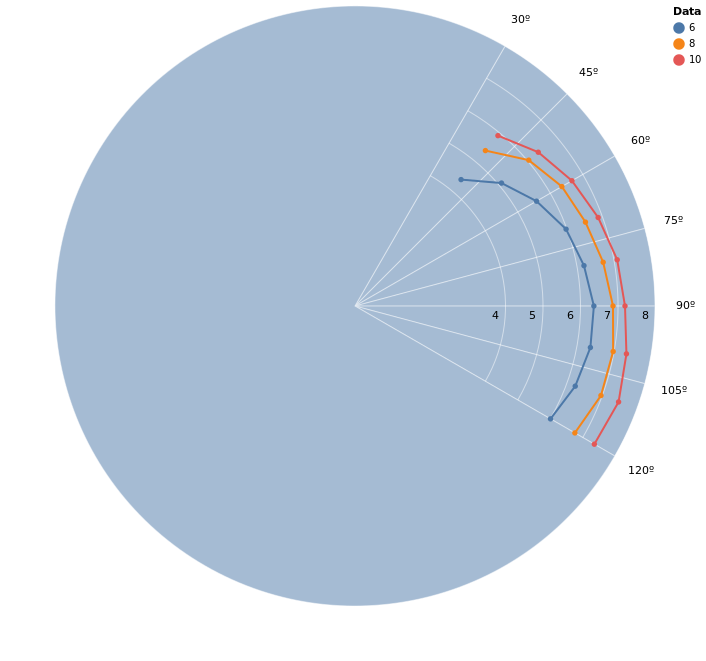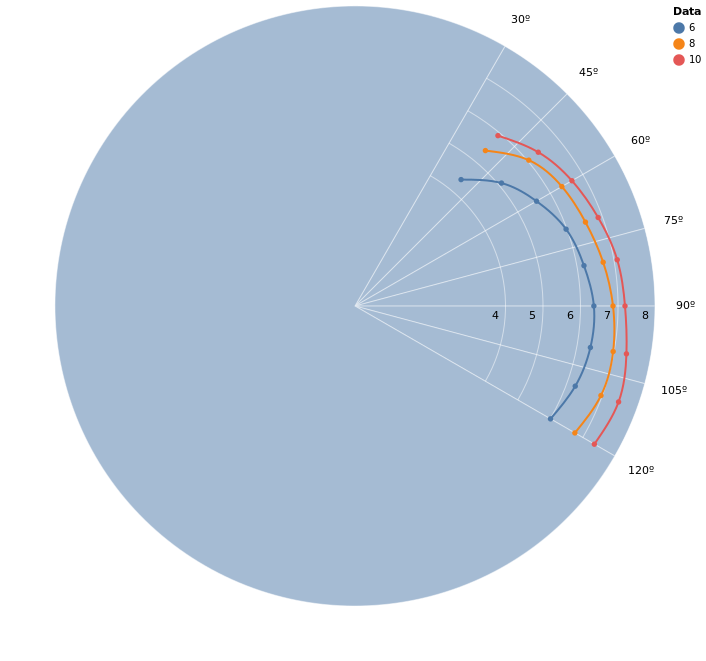# Creating Polar Plots in Elixir Part 1Software Engineer

Paulo Valente

## Introduction

In this article, we are going to analyze ORC data for sailboats, and look into how we can create polar plots in Elixir through VegaLite.

## What exactly is polar data?

We’ll use Brian’s sailboat, LittleWing, as the example data set. LittleWing is a Beneteau First 24 SE. A few years ago Brian had his boat measured and some predictive performance data generated based upon those measurements. In sailing these are called “Polars” as they’re mapped on a polar coordinate system and will show how fast the boat should go for a given wind speed and angle of attack. However, these datasets are typically sparse and don’t contain a comprehensive range of values. Below we have a table from LittleWing’s polar package that represents the boat’s speed potential given the wind speed (True Wind Speed, or TWS) and the angle of attack with respect to the wind direction (True Wind Angle, or TWA).

TWS by TWA40º50º60º70º80º90º100º110º120º
6kts4.45.15.595.996.206.376.3746.256.02
8kts5.416.056.376.546.726.886.996.986.77
10kts5.936.386.686.97.17.27.357.487.37

The fact that we have multiple functions that relate a value to an angle (3, in the example above) tells us that we’re looking at multiple sets of polar data. A polar plot is a circular plot in which each point is represented by an angle (commonly called theta, the greek alphabet letter) and an absolute distance (commonly called r, for radius).

## How to plot polar data

Now that we have a dataset in our hands, we can use VegaLite to visualize it. For this, we need to deal with the fact that the underlying `vega-lite` JS library doesn’t support polar plots. It does, however, support arc plots and normal cartesian line plots separately. We can also take advantage of the layering concept it exposes to build our custom polar plot visualization.

First, we need to install the dependencies:

``````Mix.install([
{:kino_vega_lite, "~> 0.1.3"},
{:jason, "~> 1.2"}  # Jason is needed for the SVG export we'll use.
])
```

```

### Creating the grid

With the dependencies installed, we can start creating our `PolarPlot` module which will handle the grid generation and data plotting. First, let’s take a look into how we generate the polar grid.

``````defmodule PolarPlot do
alias VegaLite, as: Vl

def plot_grid(opts \\ []) do
opts =
Keyword.validate!(opts, [
:height,
:width,
:color,
angle_marks: [0, 90, 180, 270],
theta_offset: 0,
opacity: 0.5,
stroke_color: "white",
stroke_opacity: 1
])

width = height = max(opts[:height], opts[:width])

grid_layers = angle_layers(opts)

Vl.new(height: height, width: width)
|> Vl.data_from_values(%{_r: })
end
```

```

The `plot_grid` function receives a keyword list that configures the plot. This module uses the `Keyword.validate!` function from Elixir 1.13 to ensure the options are passed correctly.

Then, we can take a look at our `angle_layers` and `radius_layers` functions separately.

``````  defp rad(angle), do: angle * :math.pi() / 180

defp angle_layers(opts) do
angle_marks_input = opts[:angle_marks]
theta_offset = opts[:theta_offset]

angle_marks = [0 | Enum.sort(angle_marks_input)] |> Enum.map(fn x -> x + theta_offset end)
angle_marks2 = tl(angle_marks) ++ [360 + theta_offset]

[angle_marks, angle_marks2]
|> Enum.zip_with(fn [t, t2] ->
label =
if t != 0 or (t == 0 and 0 in angle_marks_input) do
Vl.new()
|> Vl.mark(:text,
text: to_string(t - theta_offset) <> "º",
radius: [expr: "min(width, height) * 0.55"]
)
else
[]
end

[
Vl.new()
|> Vl.mark(:arc,
stroke: opts[:stroke_color],
stroke_opacity: opts[:stroke_opacity],
opacity: opts[:opacity],
color: opts[:color]
),
label
]
end)
|> List.flatten()
end
```

```

The `angle_layers` function utilizes the angle marks parameters as well as the plot’s graphical radius (inferred from the width and height of the plot) to plot a sequence of arcs that span a full circle, but stops at each entry in the `angle_marks` option. This will yield a graph with radial strokes.

``````  defp radius_layers(opts) do

{theta, theta2} = Enum.min_max(opts[:angle_marks])

Vl.mark(Vl.new(), :arc,
theta: theta |> rad() |> to_string(),
theta2: theta2 |> rad() |> to_string(),
color: opts[:stroke_color],
opacity: opts[:stroke_opacity]
)
end)

Vl.new()
|> Vl.data_from_values(%{
theta: Enum.map(radius_marks, fn _ -> :math.pi() / 4 end)
})
|> Vl.mark(:text,
color: "black",
theta: :math.pi() / 2,
dy: 10,
dx: -10
)
|> Vl.encode_field(:text, "r", type: :quantitative)
]

end
```

```

The `radius_marks` function serves two purposes. The first one is to plot concentric arcs of width 1 (represented in the variable `radius_marks_vl`). These arcs will serve as the radial dimension grid. The second purpose is that this function will also label said arcs, marking the values which each one represents.

This all means that the `plot_grid` function takes a base layer that marks the angles and subsequent layers that mark the radii to build a radial plot. Next, we’ll understand how `plot_data` uses the cartesian plot to plot lines over this existing grid.

### Plotting the data

We can obtain our grid base layer by calling `grid_vl = PolarPlot.plot_grid(r, opts)`. Then, we can pass that layer into the `plot_data` function to generate our final polar plot. To understand how the function works, one needs to understand that we can transform rectangular (cartesian) coordinates into polar coordinates and vice versa. The base formula for converting `(r, theta)` into `(x, y)` is `x = r.cos(theta)` and `y = r.sin(theta)`.

The main highlights in the function below are that we calculate `x_linear` and `y_linear` with the aforementioned formula, and then calculate `x` and `y` using the 2D rotation matrix to account for the `theta_offset` option.

It is also important to note that we turn off all of the labels, axes, and grid so that only the plot itself is generated. Also, the `scale` is such that the origin is placed at the center of the generated (hidden) grid, so that it coincides with the polar grid’s center. Finally, the `data` parameter is a list of tuples that contain the `r` and `theta` data for a given plot as well as the `mark` type and `mark_options` that should be used. This will become useful for plotting interpolated data, which we’ll look into in a future post. The `:grouping` and `:legend_name` options are used so we can group multiple `data` entries with the same color and to set the legend title accordingly.

``````def plot_data(vl, data, opts \\ []) do
pi = :math.pi()
legend_name = opts[:legend_name] || ""

theta_offset = opts[:theta_offset] || 0

Vl.new()
|> Vl.config(style: [cell: [stroke: "transparent"]])
|> Vl.layers([
vl
| for {r_in, theta_in, mark, mark_opts} <- data do
grouping = mark_opts[:grouping]

Vl.new()
|> Vl.data_from_values(%{
:r => r_in,
:theta => theta_in,
legend_name => List.duplicate(grouping, length(theta_in))
})
|> Vl.transform(calculate: "datum.r * cos(datum.theta * #{pi / 180})", as: "x_linear")
|> Vl.transform(calculate: "datum.r * sin(datum.theta * #{pi / 180})", as: "y_linear")
|> Vl.transform(
calculate:
as: "x"
)
|> Vl.transform(
calculate:
as: "y"
)
|> Vl.mark(mark, mark_opts)
|> Vl.encode_field(:color, legend_name, type: :nominal)
|> Vl.encode_field(:x, "y",
type: :quantitative,
scale: [
],
axis: [
grid: false,
ticks: false,
domain_opacity: 0,
labels: false,
title: false,
domain: false,
offset: 50
]
)
|> Vl.encode_field(:y, "x",
type: :quantitative,
scale: [
],
axis: [
grid: false,
ticks: false,
domain_opacity: 0,
labels: false,
title: false,
domain: false,
offset: 50
]
)
|> Vl.encode_field(:order, "theta")
end
])
end
```

```

### Plotting our first polar graph

Now that we understand the `PolarPlot` module we created, we can use it to plot the data we analyzed before.

The code below plots the data in the table above and saves it to an SVG file

``````opts = [
theta_offset: 0,
width: 600,
height: 600,
opacity: 0.5,
stroke_color: "white",
stroke_opacity: 0.5,
angle_marks: [30, 45, 60, 75, 90, 105, 120],
radius_marks: [4, 5, 6, 7, 8]
]

theta = Enum.to_list(40..120//10)

r6 = [4.4  , 5.1 , 5.59, 5.99, 6.20,  6.37, 6.374, 6.25, 6.02]
r8 = [5.41, 6.05, 6.37, 6.54, 6.72, 6.88, 6.99, 6.98, 6.77]
r10 = [5.93, 6.38, 6.68, 6.9, 7.1, 7.2, 7.35, 7.48, 7.37]

vl_grid = PolarPlot.plot_grid(opts)

data = [
{r6, theta, :line, grouping: 6, point: true},
{r8, theta, :line, grouping: 8, point: true},
{r10, theta, :line, grouping: 10, point: true}
]

vl_data = PolarPlot.plot_data(vl_grid, data, [{:stroke_opacity, 1} | opts])

svg_contents = VegaLite.Export.to_svg(vl_data)
File.write("/tmp/plot.svg", svg_contents)

vl_data
```

```We can see that because we only have a handful of points and the functions a plotted in a hidden cartesian grid, the graph just plot’s straight lines between points instead of curves we would expect in a polar plot. We can improve on that through the `:interpolate` mark option:

``````...
data = [
{r6, theta, :line, grouping: 6, point: true, interpolate: "natural"},
{r8, theta, :line, grouping: 8, point: true, interpolate: "natural"},
{r10, theta, :line, grouping: 10, point: true, interpolate: "natural"}
]
...
```

```Now we have a graph that’s closer to what we would expect. However, this doesn’t let us retrieve the values of the smooth function nor can we extrapolate the data at the edges. This is where interpolation comes in. It uses algorithms that calculate inferred functions from the given points - in fact, in the next post we will be implementing the one used for the last plot.